# Independent Component Analysis ICA Adopted from Independent Component

• Slides: 37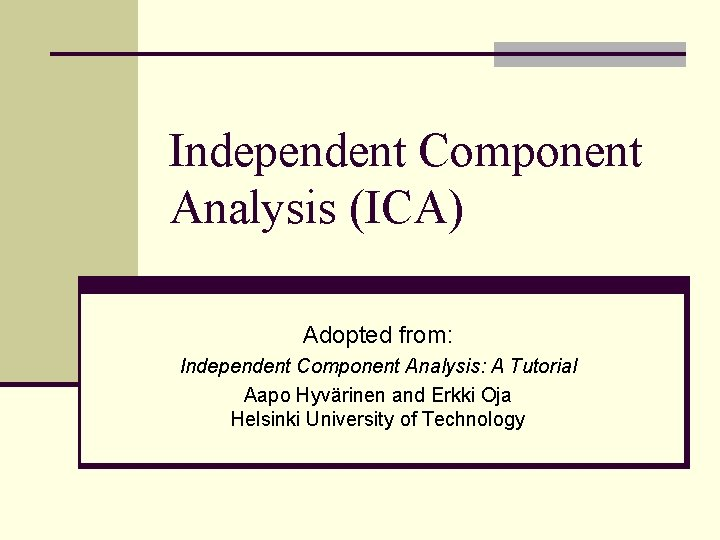Independent Component Analysis (ICA) Adopted from: Independent Component Analysis: A Tutorial Aapo Hyvärinen and Erkki Oja Helsinki University of Technology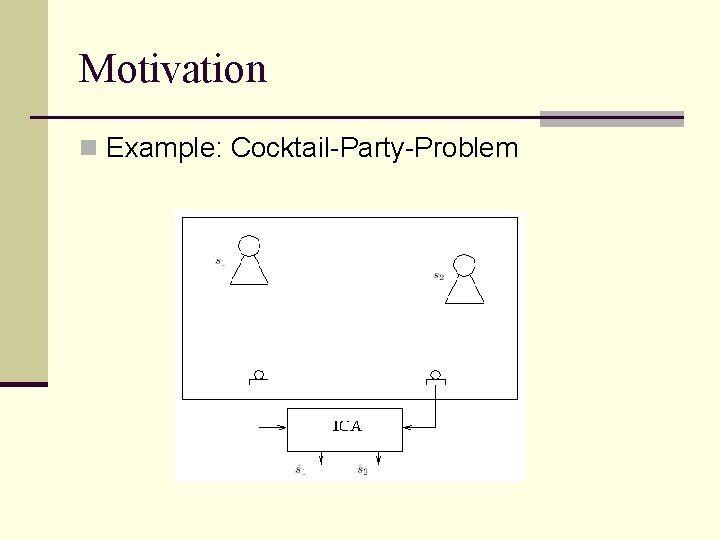Motivation n Example: Cocktail-Party-Problem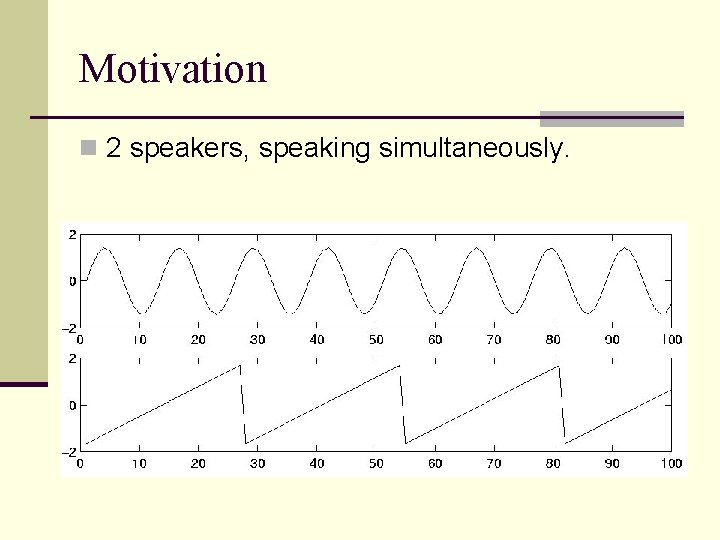Motivation n 2 speakers, speaking simultaneously.Motivation n 2 microphones in different locations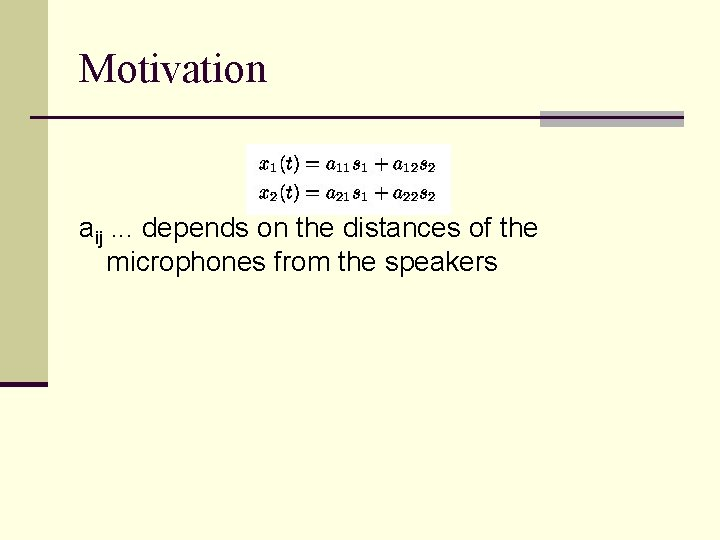Motivation aij. . . depends on the distances of the microphones from the speakers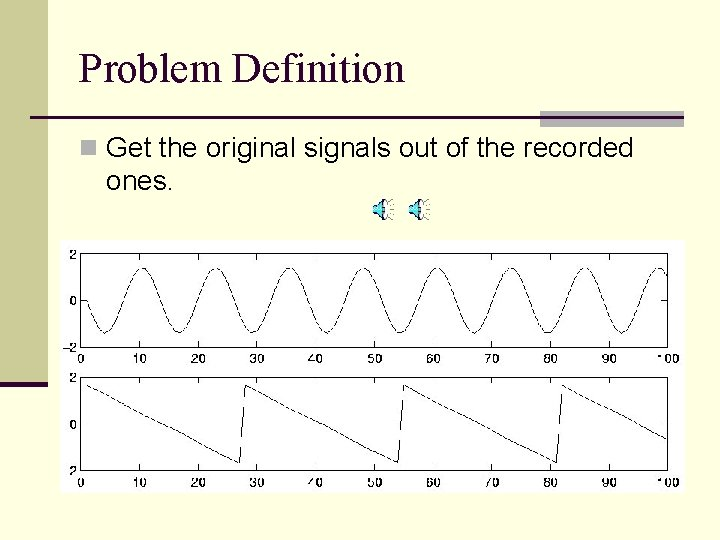Problem Definition n Get the original signals out of the recorded ones.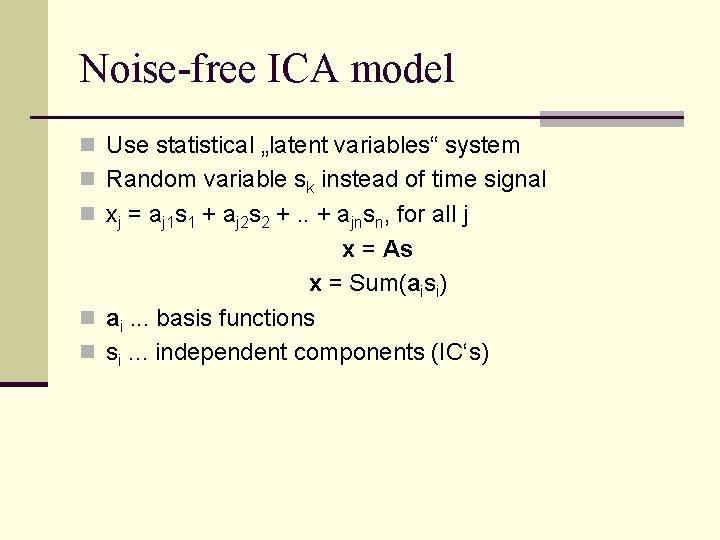Noise-free ICA model n Use statistical „latent variables“ system n Random variable sk instead of time signal n xj = aj 1 s 1 + aj 2 s 2 +. . + ajnsn, for all j x = As x = Sum(aisi) n ai. . . basis functions n si. . . independent components (IC‘s)Generative Model n IC‘s s are latent variables => unknown n Mixing matrix A is also unknown n Task: estimate A and s using only the observeable random vector xRestrictions n si are statistically independent n p(y 1, y 2) = p(y 1)p(y 2) n Non-gaussian distributions n Note: if only one IC is gaussian, the estimation is still possible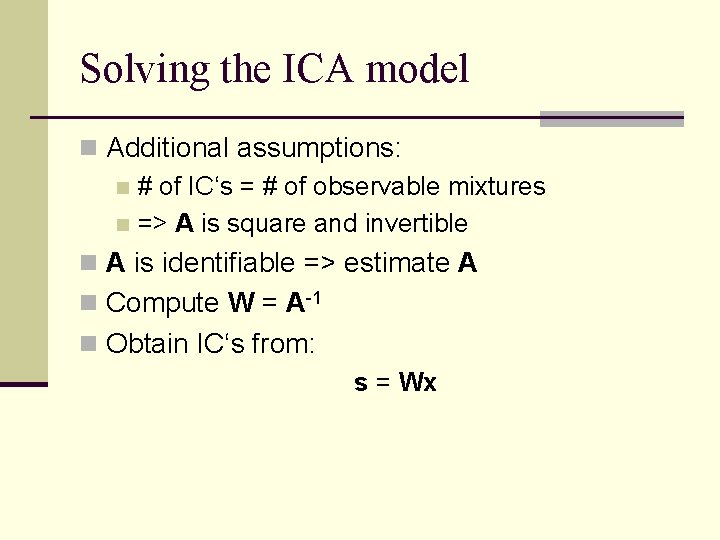Solving the ICA model n Additional assumptions: n # of IC‘s = # of observable mixtures n => A is square and invertible n A is identifiable => estimate A n Compute W = A-1 n Obtain IC‘s from: s = Wx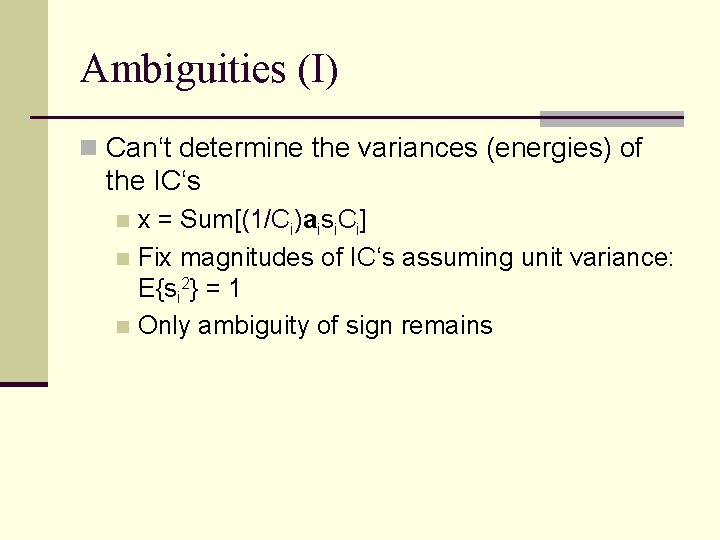Ambiguities (I) n Can‘t determine the variances (energies) of the IC‘s x = Sum[(1/Ci)aisi. Ci] n Fix magnitudes of IC‘s assuming unit variance: E{si 2} = 1 n Only ambiguity of sign remains n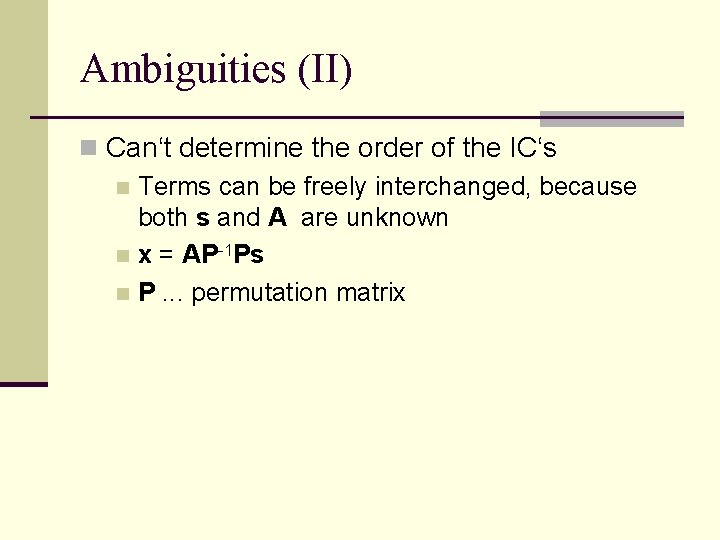Ambiguities (II) n Can‘t determine the order of the IC‘s n Terms can be freely interchanged, because both s and A are unknown n x = AP-1 Ps n P. . . permutation matrix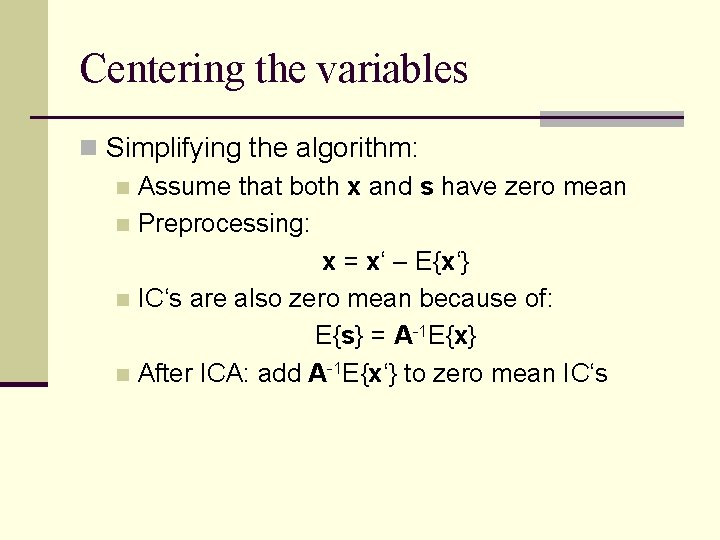Centering the variables n Simplifying the algorithm: n Assume that both x and s have zero mean n Preprocessing: x = x‘ – E{x‘} n IC‘s are also zero mean because of: E{s} = A-1 E{x} n After ICA: add A-1 E{x‘} to zero mean IC‘s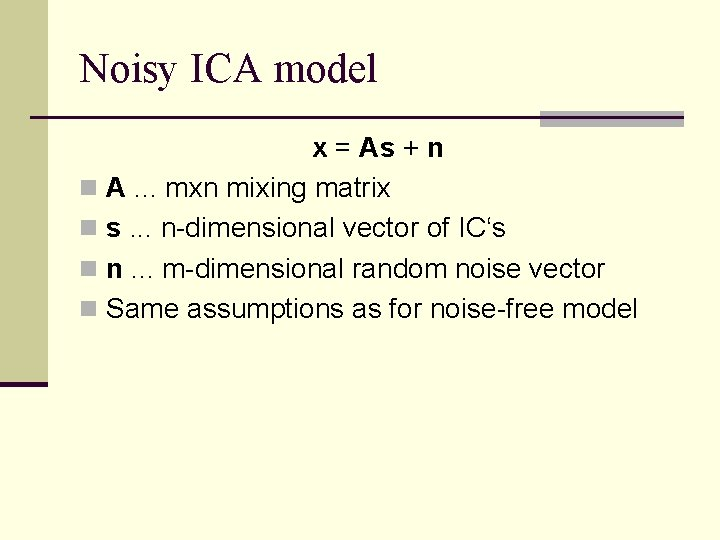Noisy ICA model x = As + n n A. . . mxn mixing matrix n s. . . n-dimensional vector of IC‘s n n. . . m-dimensional random noise vector n Same assumptions as for noise-free model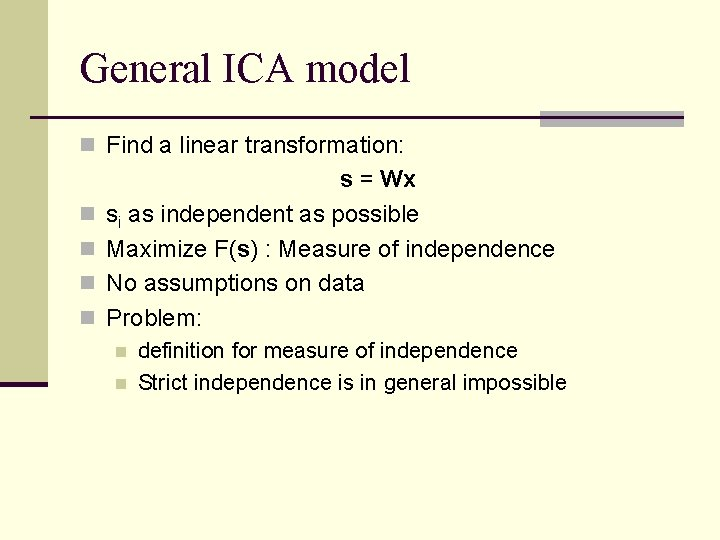General ICA model n Find a linear transformation: n n s = Wx si as independent as possible Maximize F(s) : Measure of independence No assumptions on data Problem: n n definition for measure of independence Strict independence is in general impossibleIllustration (I) n 2 IC‘s with distribution: n zero mean and variance equal to 1 n Joint distribution of IC‘s:Illustration (II) n Mixing matrix: n Joint distribution of observed mixtures: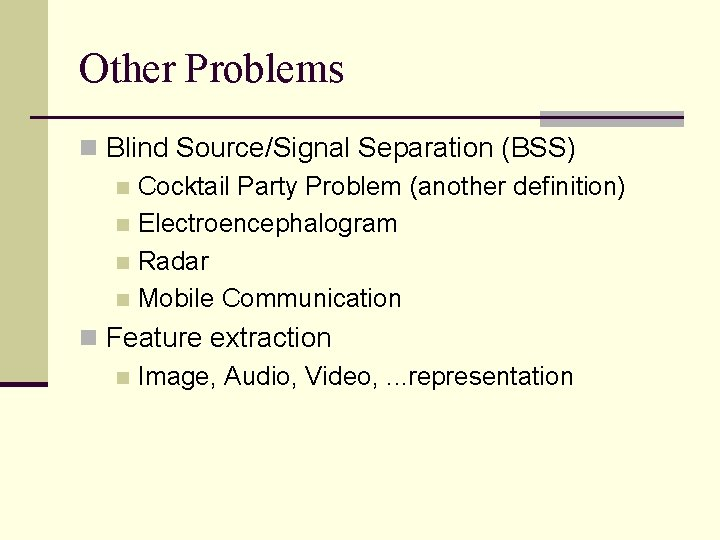Other Problems n Blind Source/Signal Separation (BSS) n Cocktail Party Problem (another definition) n Electroencephalogram n Radar n Mobile Communication n Feature extraction n Image, Audio, Video, . . . representation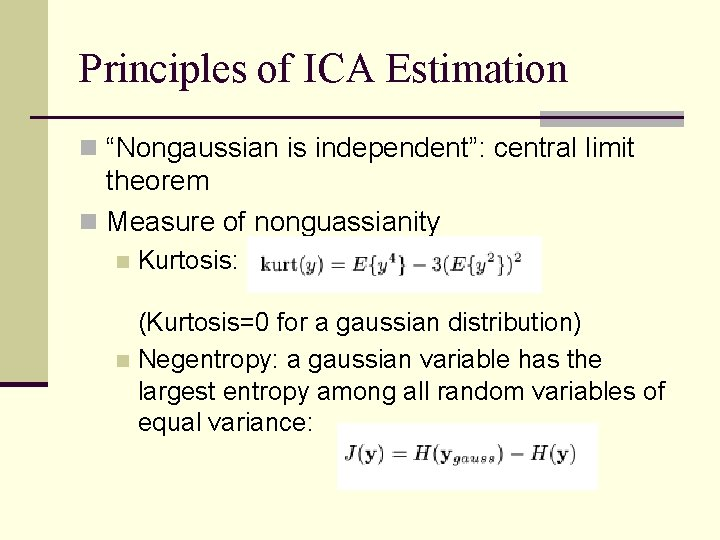Principles of ICA Estimation n “Nongaussian is independent”: central limit theorem n Measure of nonguassianity n Kurtosis: (Kurtosis=0 for a gaussian distribution) n Negentropy: a gaussian variable has the largest entropy among all random variables of equal variance: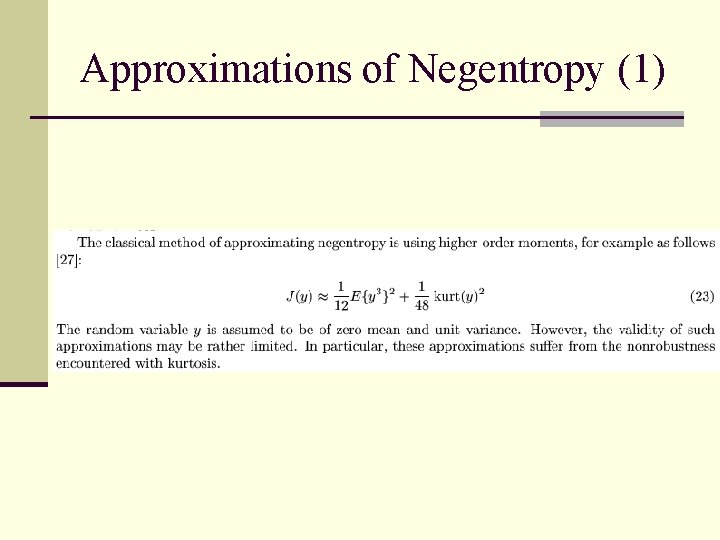Approximations of Negentropy (1)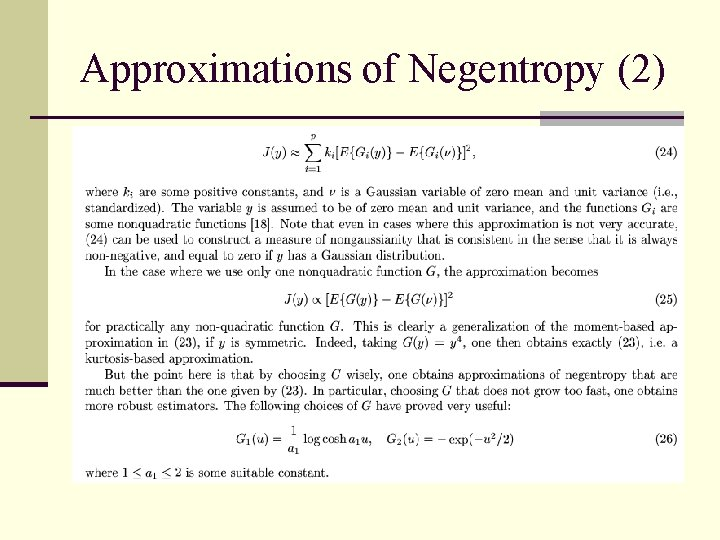Approximations of Negentropy (2)The Fast. ICA Algorithm4 Signal BSS demo (original)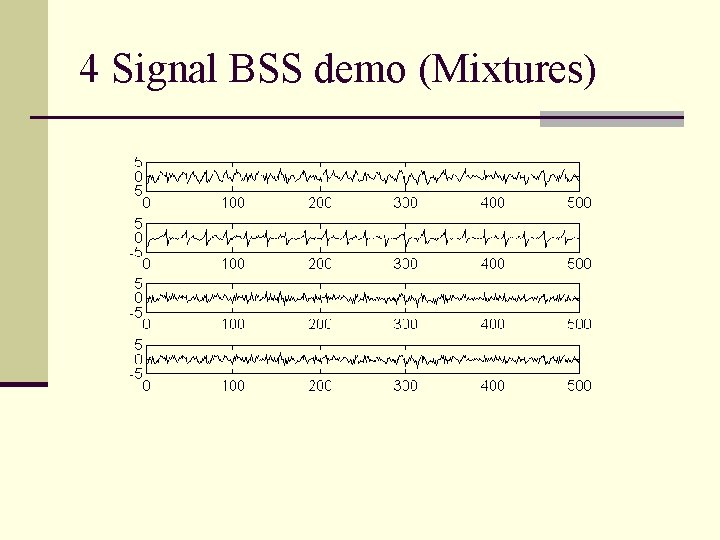4 Signal BSS demo (Mixtures)4 Signal BSS demo (ICA)Fast. ICA demo (mixtures)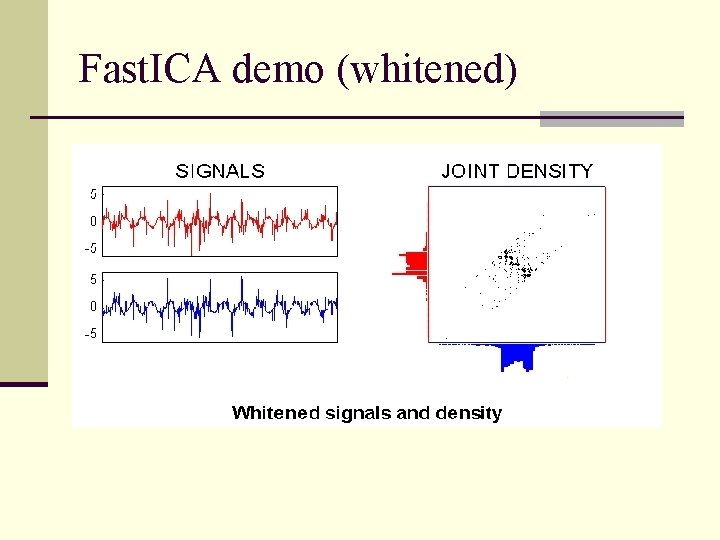Fast. ICA demo (whitened)Fast. ICA demo (step 1)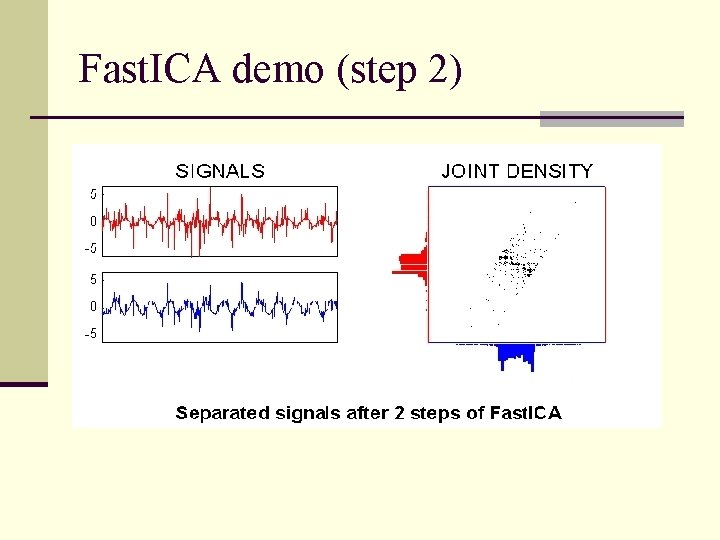Fast. ICA demo (step 2)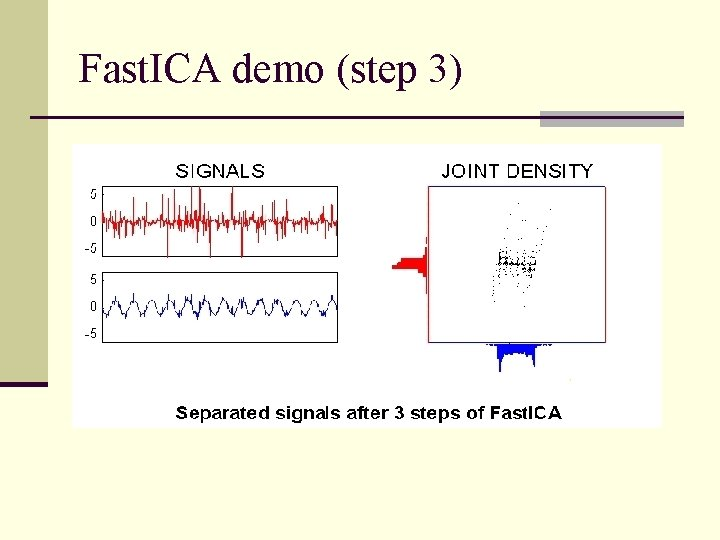Fast. ICA demo (step 3)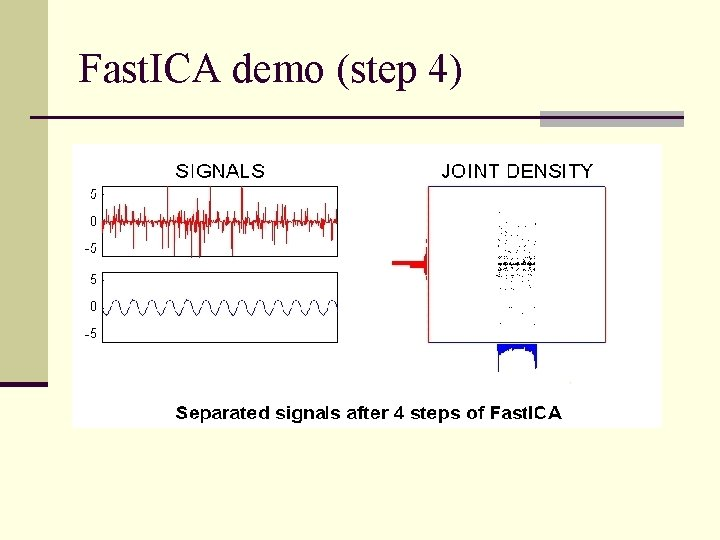Fast. ICA demo (step 4)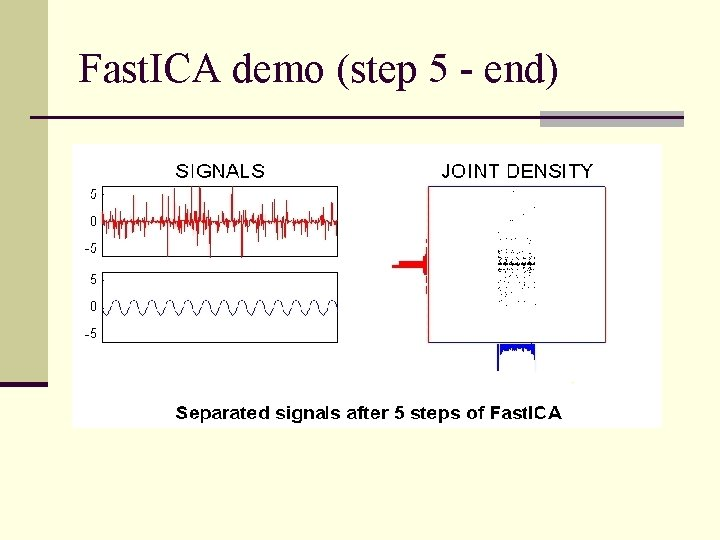Fast. ICA demo (step 5 - end)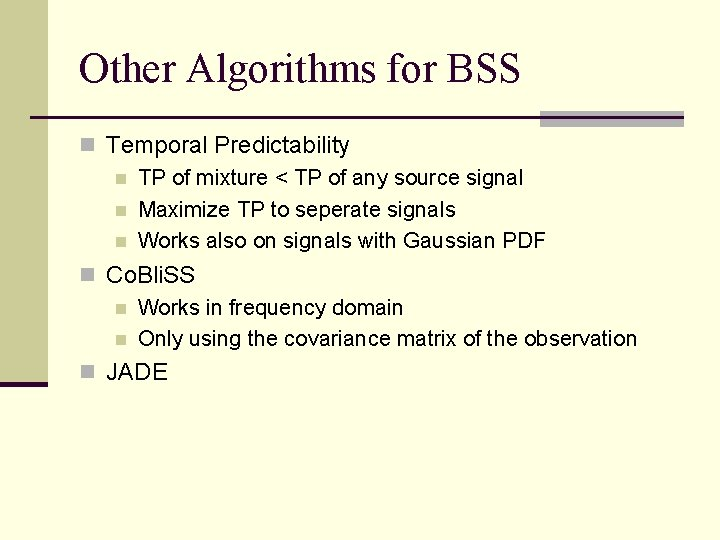Other Algorithms for BSS n Temporal Predictability n TP of mixture < TP of any source signal n Maximize TP to seperate signals n Works also on signals with Gaussian PDF n Co. Bli. SS n Works in frequency domain n Only using the covariance matrix of the observation n JADELinks 1 n Feature extraction (Images, Video) n http: //hlab. phys. rug. nl/demos/ica/ n Aapo Hyvarinen: ICA (1999) n http: //www. cis. hut. fi/aapo/papers/NCS 99 web/node 11. ht ml n ICA demo step-by-step n http: //www. cis. hut. fi/projects/icademo/ n Lots of links n http: //sound. media. mit. edu/~paris/ica. html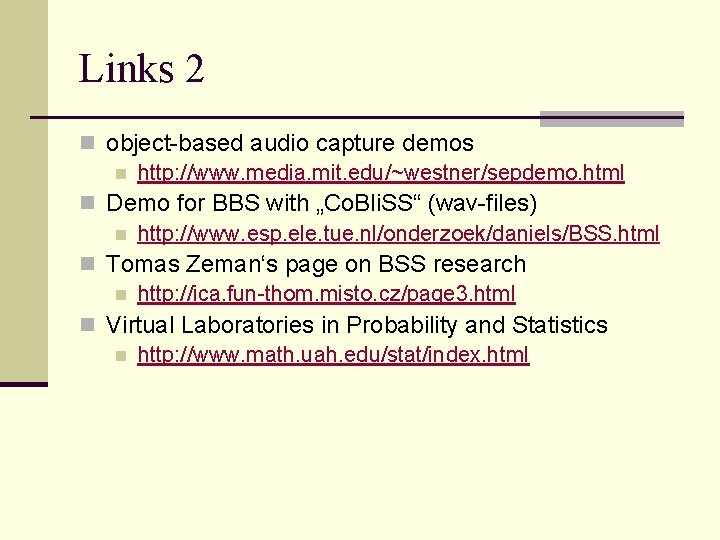Links 2 n object-based audio capture demos n http: //www. media. mit. edu/~westner/sepdemo. html n Demo for BBS with „Co. Bli. SS“ (wav-files) n http: //www. esp. ele. tue. nl/onderzoek/daniels/BSS. html n Tomas Zeman‘s page on BSS research n http: //ica. fun-thom. misto. cz/page 3. html n Virtual Laboratories in Probability and Statistics n http: //www. math. uah. edu/stat/index. html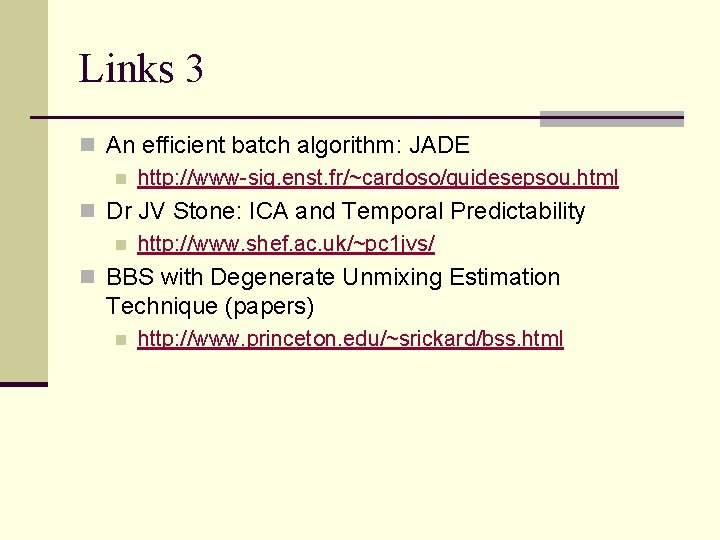Links 3 n An efficient batch algorithm: JADE n http: //www-sig. enst. fr/~cardoso/guidesepsou. html n Dr JV Stone: ICA and Temporal Predictability n http: //www. shef. ac. uk/~pc 1 jvs/ n BBS with Degenerate Unmixing Estimation Technique (papers) n http: //www. princeton. edu/~srickard/bss. html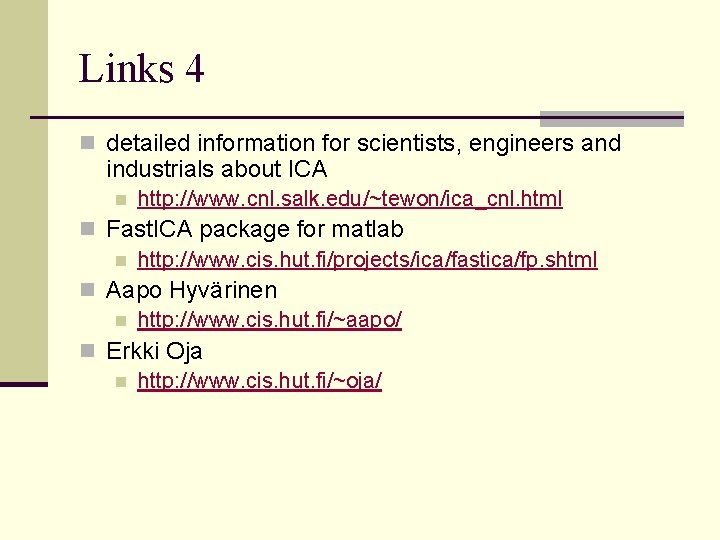Links 4 n detailed information for scientists, engineers and industrials about ICA n http: //www. cnl. salk. edu/~tewon/ica_cnl. html n Fast. ICA package for matlab n http: //www. cis. hut. fi/projects/ica/fastica/fp. shtml n Aapo Hyvärinen n http: //www. cis. hut. fi/~aapo/ n Erkki Oja n http: //www. cis. hut. fi/~oja/